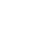# How to make a digital clock using JavaScript

Last modified March 28th 2022 | #js |Source Code [GitHub]

In this tutorial we’ll be building a digital clock using JavaScript.

Let’g get started by creating a `<div>` element that’ll be used to display the time:

```.wp-block-code {
border: 0;
}

.wp-block-code > div {
overflow: auto;
}

.shcb-language {
border: 0;
clip: rect(1px, 1px, 1px, 1px);
-webkit-clip-path: inset(50%);
clip-path: inset(50%);
height: 1px;
margin: -1px;
overflow: hidden;
position: absolute;
width: 1px;
word-wrap: normal;
word-break: normal;
}

.hljs {
box-sizing: border-box;
}

.hljs.shcb-code-table {
display: table;
width: 100%;
}

.hljs.shcb-code-table > .shcb-loc {
color: inherit;
display: table-row;
width: 100%;
}

.hljs.shcb-code-table .shcb-loc > span {
display: table-cell;
}

.wp-block-code code.hljs:not(.shcb-wrap-lines) {
white-space: pre;
}

.wp-block-code code.hljs.shcb-wrap-lines {
white-space: pre-wrap;
}

.hljs.shcb-line-numbers {
border-spacing: 0;
counter-reset: line;
}

.hljs.shcb-line-numbers > .shcb-loc {
counter-increment: line;
}

.hljs.shcb-line-numbers .shcb-loc > span {
}

.hljs.shcb-line-numbers .shcb-loc::before {
border-right: 1px solid #ddd;
content: counter(line);
display: table-cell;
text-align: right;
-webkit-user-select: none;
-moz-user-select: none;
-ms-user-select: none;
user-select: none;
white-space: nowrap;
width: 1%;
}
```const clock = document.createElement("div");
document.body.appendChild(clock);```Code language: JavaScript (javascript)```

Next create a `getTime` function that’ll calculate the time:

``````const getTime = () => {
const date = new Date();
let hour = date.getHours();
let min = date.getMinutes();
let sec = date.getSeconds();
}```Code language: JavaScript (javascript)```

We’ve used JavaScripts built in `Date()` object here which represents a single moment in time. From this we then extract the hours, minutes, and seconds. Each measurement is assigned it’s own variable as we’ll need to do some manipulation in the next step.

Hours, minutes, and seconds less than 10 don’t have a leading zero digit which is commonly used when formatting digital time. To achieve this we’ll check if the unit of measure is less than 10. If it is we’ll prepend a zero otherwise the measurement is left alone:

```.hljs > mark.shcb-loc { background-color: #4D525C; }```const getTime = () => {
const date = new Date();
let hour = date.getHours();
let min = date.getMinutes();
let sec = date.getSeconds();
hour = (hour < 10) ? "0" + hour : hour;
min = (min < 10) ? "0" + min : min;
sec = (sec < 10) ? "0" + sec : sec;
};
```Code language: JavaScript (javascript)```

We can now insert the time to our clock `<div>`:

``````const getTime = () => {
const date = new Date();
let hour = date.getHours();
let min = date.getMinutes();
let sec = date.getSeconds();
hour = ("0" + hour).slice(-2);
min = ("0" + min).slice(-2);
sec = ("0" + sec).slice(-2);
const time = hour + ":" + min + ":" + sec;
clock.innerText = time;
};
```Code language: JavaScript (javascript)```

If you were to run the `getTime` function at this point it would fetch the current time at execution. As we’re building a digital clock we’ll need to fetch and update the time using the `SetInterval` method every second (1000 milliseconds):

``````const getTime = () => {
const date = new Date();
let hour = date.getHours();
let min = date.getMinutes();
let sec = date.getSeconds();
hour = ("0" + hour).slice(-2);
min = ("0" + min).slice(-2);
sec = ("0" + sec).slice(-2);
const time = hour + ":" + min + ":" + sec;
clock.innerText = time;
};
setInterval(getTime, 1000);
```Code language: JavaScript (javascript)```

Currently the clock is displaying time in the 24 hour format. To convert to a 12 hour format clock we just need to check if the current hour is greater than 12, if true subtract 12 hours. We’ll also declare a merdiem variable and assign it either a AM or PM which will get displayed after the time:

``````const getTime = () => {
const date = new Date();
let hour = date.getHours();
let min = date.getMinutes();
let sec = date.getSeconds();
hour = ("0" + hour).slice(-2);
min = ("0" + min).slice(-2);
sec = ("0" + sec).slice(-2);
let meridiem;
if (hour > 12) {
meridiem = "PM";
hour = hour - 12;
} else {
meridiem = "AM";
}
const time = hour + ":" + min + ":" + sec + " " + meridiem;
clock.innerText = time;
};
```Code language: JavaScript (javascript)```

You should now have a fully functioning digital clock built using JavaScript. When it comes to styling this clock it would be best to use a monospace font (`font-family: monospace;`). This will prevent the clock dimensions shifting due to the variable font width between the different numbers.